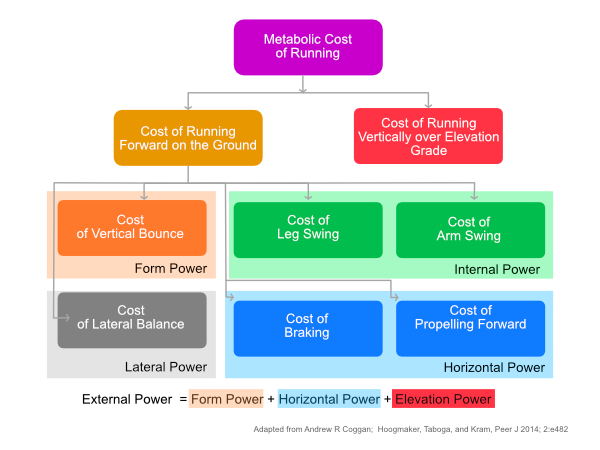# Geeks on Feet

for the love of running

## The Science Behind Running Power

A primer on the science behind running power and how Stryd power meter measures running powerSeveral runners have approached me with questions related to how running power is calculated, after my previous posts on Running Power (Part1, Part2). This post goes into the details of running power, and the science behind it.

### Running Power Defined

Power is the mechanical measure of running effort and intensity. Power indicates how much energy you are expending during the run x how fast you are expending it.

Let us quantify this definition through a series of comprehensible formulae:

Power is determined by calculating the amount of work done per unit of time

Power = Work ÷ Time

Work is equal to the force applied multiplied by the distance run

Work = Force x Distance

Thus, power can also be expressed as …

Power = (Force x Distance) ÷ Time

Yeah, this is getting a little too mathematical, but just bear with me for a tad bit more 😉 re-aligning the braces above we get …

Power = Force x (Distance ÷ Time)

Now, this is the power equation when you talk about power-meters in the context of cycling. On a bike, power is determined by multiplying the force applied to the pedals and the rate at which the pedals turn aka Cadence. However, running power meters function differently. We can refine the power equation (one last time) to break it down further …

Power = Mass x Acceleration x Velocity

### How does Stryd calculate Running power?

As per the above equation on Power, Stryd takes in the mass (or weight) of you and your acceleration and speed to calculate power, measured in Watts.

Before we can understand how Stryd calculates power, it is important to understand how we actually expend energy when running. When running we move different parts of our body to move body’s Centre of Mass (COM) forward. We move our arms & legs, we bounce from the ground, we brake, we balance, and more. All of these actions need energy, and this is called the ‘Metabolic cost of running’.

The following diagram illustrates the various components that make-up metabolic cost.Exeternal Power and components that make-up Metabolic

The power that Stryd measures(Stryd Power) only represents the External Power in the diagram; that is the metabolic cost of moving ourselves forward. Stryd doesn’t measure Internal power, which is the metabolic cost of swinging our arms, legs and other movements that don’t directly contribute to moving us forward.

Stryd Power(External Power) = Horizontal Power + Form Power + Elevation Power

• Stryd’s Form Power is the metabolic cost of oscillating our COM up and down vertically (vertical bounce) as we move forward.
• Horizontal Power is the metabolic cost of moving the COM forward horizontally, or longitudinally along the line of progression.
• Elevation power is the metabolic cost of elevating the COM vertically up a hill or incline.

As you can expect, on a flat terrain the elevation power is zero. So in such case,

Stryd Power = Horizontal Power + Form Power

Form Power doesn’t contribute to our running speed and correlates negatively with speed. Horizontal Power correlates positively with speed. So improving Horizontal Power is an important component of improving speed. Horizontal power is a power metric that one can track and see how they are improving their power over time.

### Instantaneous power and average power

When running outdoors on the road (or trail) power is rather stochastic as illustrated below

Because power is stochastic in nature. It is helpful to analyze a power file from the perspective of averages. In a way, this is like setting a watch to display 10s rolling average power, the 30s rolling average power, or even lap power.

Stryd supports the following power averaging metrics:

• Real-Time Power: The second by second power from Stryd
• 3 Second Power
• 10 Second Power
• 30 Second Power: The last 3, 10, and 30 Second averaged power from Stryd
• Lap Power: The averaged power between the start of the lap and now
• Overall Power: The averaged power across the entire activity

For the technically inclined readers who want to explore the Stryd algorithm, I suggest this excellent post by Ron George.Kartik Iyer is a conversationalist, running geek, techie, marathoner, miles to go CrossFit junkie and bathroom Carnatic vocalist. He loves striking random conversations with people just about anywhere, music and anyting to do with tech and fitness, in no particular order. He can be reached at @kartikiyer2007 on Insta and on Strava